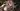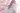# Knuth–Morris–Pratt the KMP Algorithm

2 years ago
571
2 min

## First acquaintance with BF and KMP algorithms

Firstly, let’s look at these two codes:

int BF()
{
auto pattern_size = pattern.size();
auto text_size = text.size();
auto pattern_index = 0;
auto text_index = 0;

while (pattern_index < pattern_size && text_index < text_size)
{
if (pattern[pattern_index] == text[text_index]) //success
{
++text_index;
++pattern_index;
}
else  //failure
{
text_index -= pattern_index - 1;
pattern_index = 0;
}
}
if (pattern_index == pattern_size)    return text_index - pattern_index;
return -1;
}

int KMP()
{
auto pattern_size = pattern.size();
auto text_size = text.size();
auto pattern_index = 0;
auto text_index = 0;

while (pattern_index < pattern_size && text_index < text_size)
{
if (pattern_index == -1 || pattern[pattern_index] == text[text_index])  //success
{
++text_index;
++pattern_index;
}
else    pattern_index = next[pattern_index];  //failure
}
if (pattern_index == pattern_size)    return text_index - pattern_index;
return -1;
}


The two pieces of code above are BF Algorithm and MP(KMP) Algorithm.

By observing we can see that the two pieces of code are strikingly similar.

Let’s discover the relationship between them by simulating the process performed by the algorithm.BF Algorithm is naive matching algorithm. It’s idea is to match the text string and the pattern string bit by bit from the beginning. If the match fails (mismatch), the pattern string is shifted to the right by one bit, and then matched from the beginning.

The above picture shows $13$ times matching.

It can be found that the BF Algorithm can be very efficient in the case of too many mismatches.The 2 and 4 steps in the BF Algorithm are useless matches. Because $pattern = text$ and $text \neq text$ , therefore, $pattern \neq text$. The second step must match the failure, the fourth step is the same. MP(KMP) Algorithm in order to improve this, do not backtrack the subscript of the text string, only change the subscript of the pattern string, minus the two steps.

The above picture shows $11$ times matching.

We get the first conclusion:

The difference between the MP (KMP) Algorithm and the BF Algorithm is that the pattern string is shifted to the right by one bit each time the BF Algorithm is mismatched, and the MP (KMP) Algorithm is shifted to the right by $k(k \geq 1, k \in N)$ bits.

## Calculate the number of right shifts

Knowing the difference between MP(KMP) Algorithm and BF Algorithm, the key now is how to calculate the right-shifted $k$ value.

Let‘s look at three more examples:Marking that $P$ is the set of all prefixes of the pattern string, $S$ is the set of all suffixes of the pattern string, for the matched string of the example one $P=\{A,AB,ABC,ABCA,ABCAB\}$, $S=\{B,AB,CAB,BCAB,ABCAB\}$.

It can be found that the longest same element of the P and S of the matching string is AB, and the previous two matches are to move the pattern string from the prefix AB to the suffix AB.

Since MP(KMP) Algorithm is traversed from left to right, we only need to record the length of the longest same element of the $P$ and $S$ of all prefix substrings of the pattern string as $l[i]$. And then, after each mismatch, the pattern string is shifted to the right by $Length-l[i-1]-1. Marking$next[i]=l[i]\$, and the MP(KMP) Algorithm will come out.

So we get the second conclusion:

MP Algorithm‘s right-shifted $k$ value equal to the longest same element of the $P$ and $S$ of the matched pattern string.

## Compute next array

So how do you calculate $next[i]$? The answer is still coming from MP(KMP) Algorithm.

We match the pattern string with itself, noting that the string that matches successfully is the longest same element of the $P$ and $S$ of the corresponding substring.Therefore, we only need to add the assignment statement to the $next$ array after matching in the MP(KMP) Algorithm program to complete the solution to the $next$ array. ($next=-1, next[i]=0, i > 0$)

int GetNext()
{
next = -1;
auto prefix = -1;
auto suffix = 0;
while (suffix < pattern_size - 1)
{
if (prefix == -1 || pattern[prefix] == pattern[suffix])
{
++prefix;
++suffix;
next[suffix] = next[prefix];  //assignment
}
else    prefix = next[prefix];
}
}


At this point, we have completed all the code of MP Algorithm.

The third conclusion:

Match the pattern string to itself to get the $next$ array.

## Complete KMP algorithm

Let‘s look at an example:In this example, the second match must fail. Because $pattern[i]=pattern[next[i]]$, and $pattern[i] \neq text[i]$, therefore, $pattern[next[i]] \neq text[i]$.

So we add a judgment to the function to optimize the algorithm.

int GetNext_KMP()
{
next = -1;
auto prefix = -1;
auto suffix = 0;
while (suffix < pattern_size - 1)
{
if (prefix == -1 || pattern[prefix] == pattern[suffix])
{
++prefix;
++suffix;
if (pattern[prefix] != pattern[suffix])    next[suffix] = prefix;
else    next[suffix] = next[prefix];
}
else    prefix = next[prefix];
}
}


In the end, we get all the code for KMP Algorithm.

## Time Complexity

• BF Algorithm: $O(nm)$
• MP Algorithm: $O(m)+O(n+m)$
• KMP Algorithm: $O(m)+O(n+m)$

• BF Algorithm:Brute Force Algorithm
• MP Algorithm:Morris-Pratt Algorithm
• KMP Algorithm:Knuth-Morris-Pratt Algorithm
• Reference Code
Last modified on November 3rd 2020 4:55:53 pmUse WSL to Build a Development Environment
© 2020 · YXL
Build with Gatsby